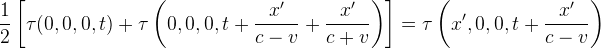## Why is Special Relativity Wrong (2)

Galileo might be wrong, Einstein could also be incorrect.

Listed below are all the possibilities:

 Galileo's formula Einstein's formula Case 1 Wrong Wrong Case 2 Correct Wrong Case 3 Wrong Correct Case 4 Correct Correct

Case 4 is not possible, because the two formulas contradict each other.

Case 1 & 2 can be omitted, as we are trying to prove that Special Relativity is wrong. If we assume Special Relativity is wrong, then no further discussion is needed.

So case 3 is the only possibility.

Does that mean Special Relativity is correct?
No, because in the first equation* of Special Relativity, the Galileo Transformation is used, or else the terms c-v and c+v would not appear:Since it is built from the wrong basis, Special Relativity cannot be correct!

*It appears as the first equation in section 3, part I, of "On the Electrodynamics of Moving Bodies", the paper on Special Relativity by Einstein.